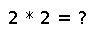pinv

``````..  pinv(M[, tol])

Computes the Moore-Penrose pseudoinverse.

For matrices ``M`` with floating point elements, it is convenient to compute
the pseudoinverse by inverting only singular values above a given threshold,
``tol``.

The optimal choice of ``tol`` varies both with the value of ``M``
and the intended application of the pseudoinverse. The default value of
``tol`` is ``eps(real(float(one(eltype(M)))))*maximum(size(A))``,
which is essentially machine epsilon for the real part of a matrix element
multiplied by the larger matrix dimension.
For inverting dense ill-conditioned matrices in a least-squares sense,
``tol = sqrt(eps(real(float(one(eltype(M))))))`` is recommended.

.. [issue8859] Issue 8859, "Fix least squares", https://github.com/JuliaLang/julia/pull/8859
.. [B96] Åke Björck, "Numerical Methods for Least Squares Problems",
SIAM Press, Philadelphia, 1996, "Other Titles in Applied Mathematics", Vol. 51.
`doi:10.1137/1.9781611971484 <http://epubs.siam.org/doi/book/10.1137/1.9781611971484>`_
.. [S84] G. W. Stewart, "Rank Degeneracy", SIAM Journal on
Scientific and Statistical Computing, 5(2), 1984, 403-413.
`doi:10.1137/0905030 <http://epubs.siam.org/doi/abs/10.1137/0905030>`_
.. [KY88] Konstantinos Konstantinides and Kung Yao, "Statistical analysis
of effective singular values in matrix rank determination", IEEE
Transactions on Acoustics, Speech and Signal Processing, 36(5), 1988,
757-763.
`doi:10.1109/29.1585 <http://dx.doi.org/10.1109/29.1585>`_``````

Examples

Checking you are not a robot: